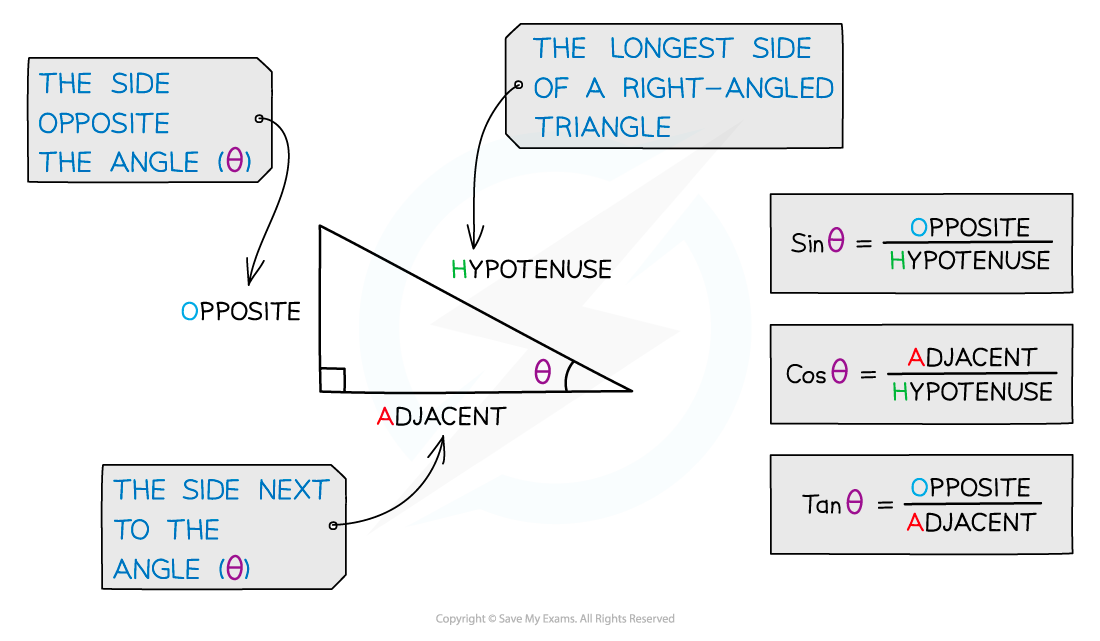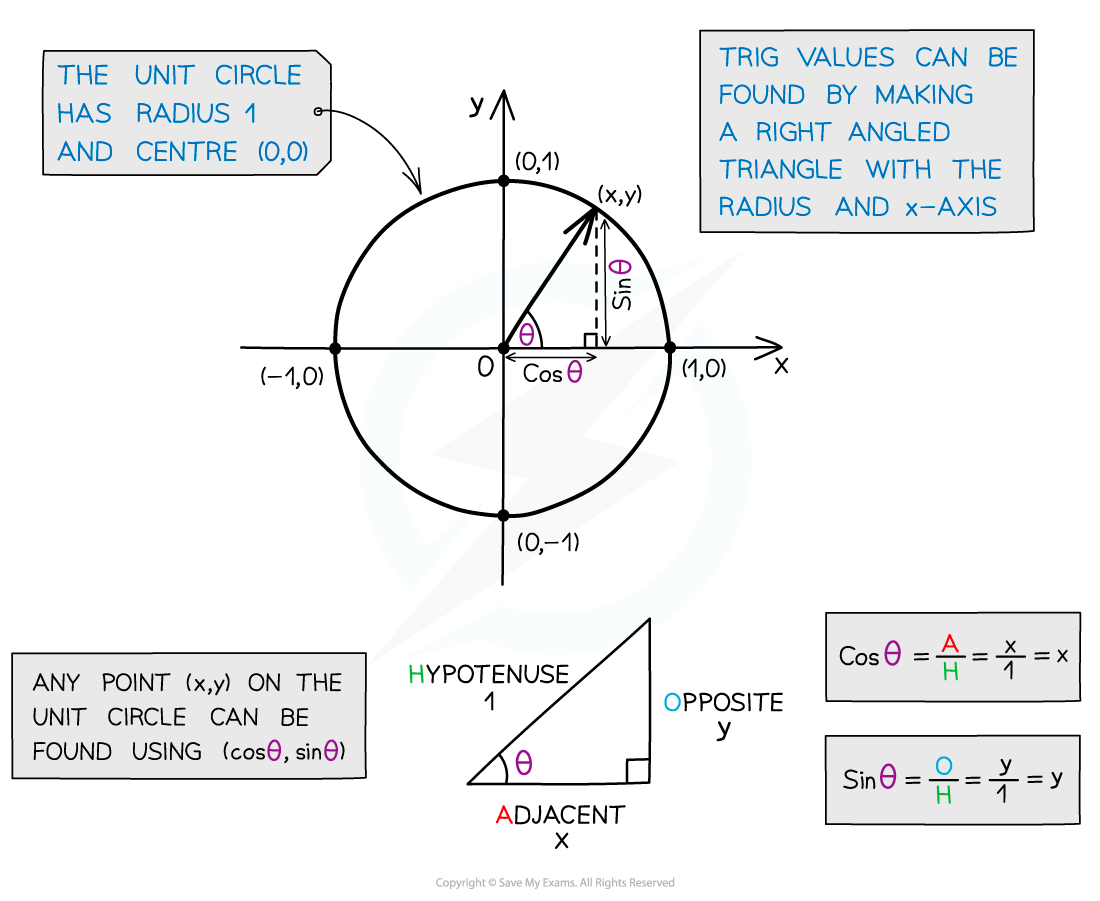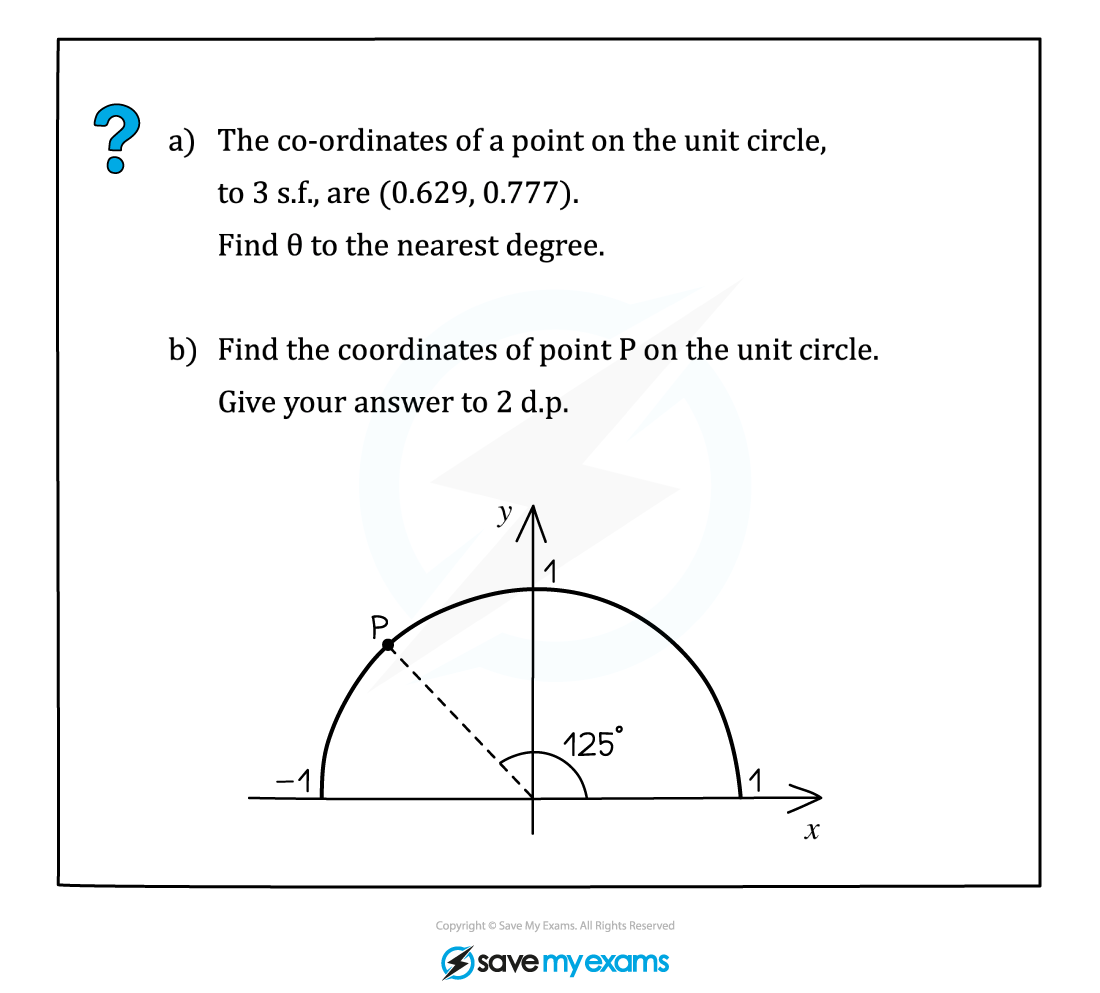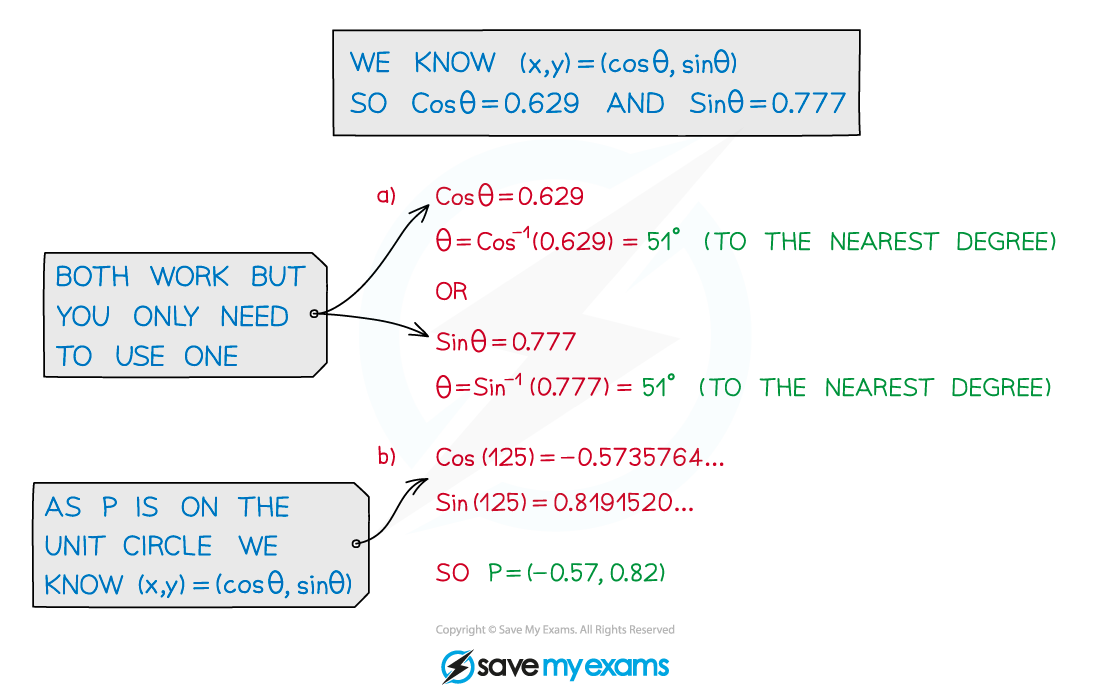# AQA A Level Maths: Pure复习笔记5.1.1 Trigonometry - Definitions

### Trigonometry - Definitions

#### What is trigonometry?

• Trigonometry looks at the relationship between side lengths and angles of triangles
• It comes from the Greek words trigonon meaning ‘triangle’ and metron meaning ‘measure’
• The three trigonometric functions Sine, Cosine and Tangent come from ratios of side lengths in right angled triangles#### What is the unit circle and how does it help with trigonometry?

• The unit circle is a circle with radius 1 and centre (0, 0)
• It can be used to calculate trig values as a co‑ordinate point (x, y) on the circle
• (x, y) = (cos θ, sin θ), where θ is the angle measured anticlockwise from the positive x‑axis
• It allows us to calculate sin, cos and tan for angles greater than 90°#### Worked Example